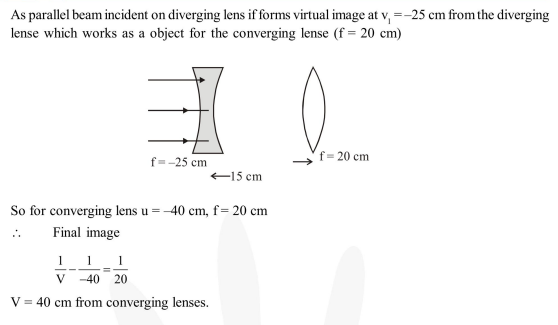Question:

A diverging lens with magnitude of focal length $25 \mathrm{~cm}$ is placed at a distance of $15 \mathrm{~cm}$ from a converging lens of magnitude of focal length $20 \mathrm{~cm}$. A beam of parallel light falls on the diverging lens. The final image formed is :

1. real and at a distance of $40 \mathrm{~cm}$ from the divergent lens

2.  real and at a distance of $6 \mathrm{~cm}$ from the conver

3. real and at a distance of $40 \mathrm{~cm}$ from convergent lens

4.  virtual and at a distance of $40 \mathrm{~cm}$ from convergent lens.

Correct Option: , 3

Solution: